17.06.2019

## Motivation

Bivariate sample: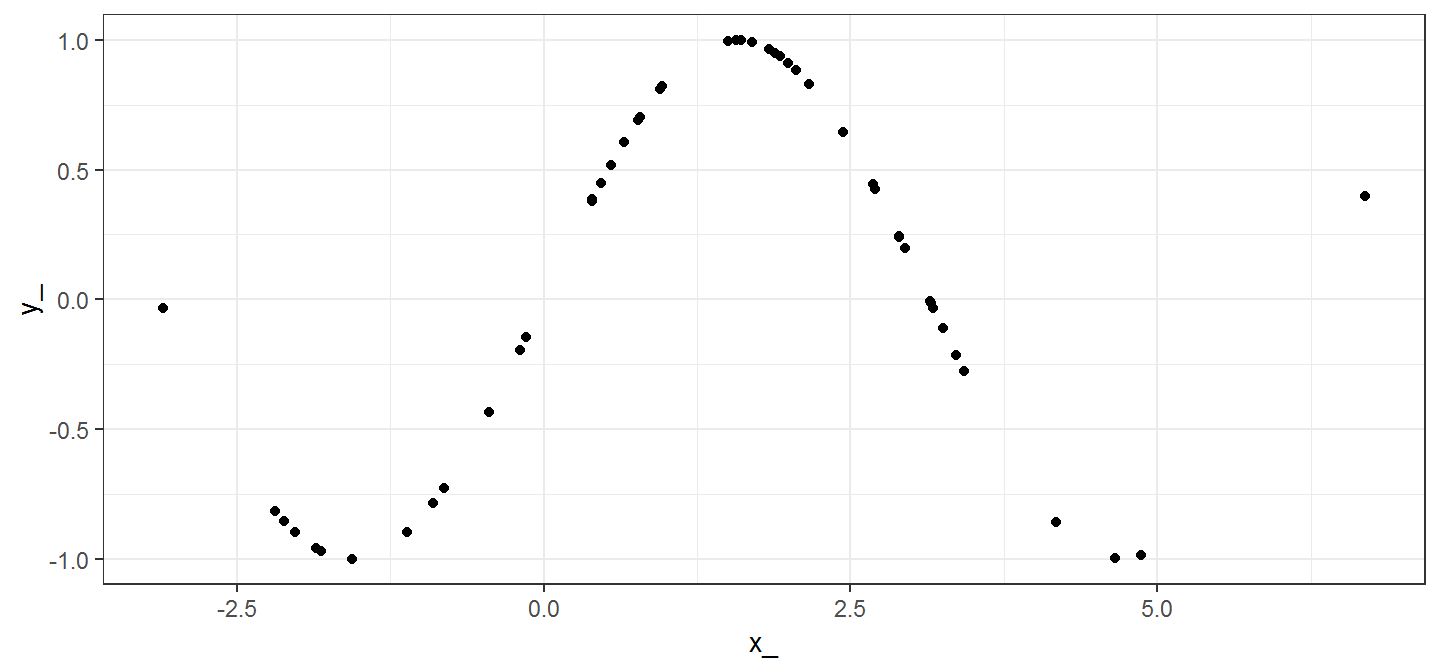$$X \sim \mathcal{N}(1.42,2)$$, $$Y = \sin(X)$$.

Would it be easier to predict $$Y$$ given $$X$$, or $$X$$ given $$Y$$?

## Motivation

Real life example: Average speed vs. Fuel consumption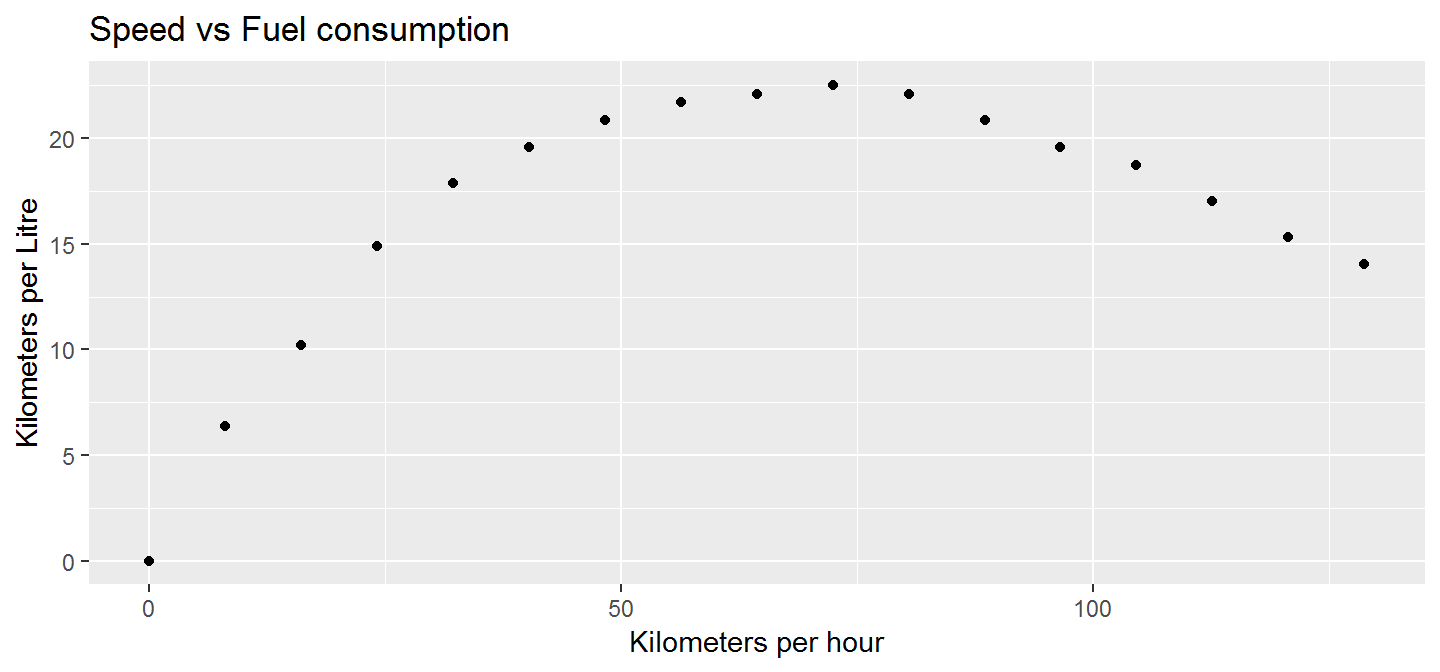## Motivation

What is dependence between random variables $$X$$ and $$Y$$?

$$X$$ …result of a coin toss,
$$Y$$ …result of tossing the same coin a second time.
-> we do not gain information about $$Y$$ if we know $$X$$ and vice versa.

$$X$$ …result of drawing a card from a deck containing 2 cards,
$$Y$$ …result of drawing the remaining card.
-> we know everything about $$Y$$ if we know $$X$$ and vice versa.

## Motivation

Why could this be a problem?

How would we usually quantify dependence?

## Motivation

Why could this be a problem?

How would we usually quantify dependence?

Correlation coefficient:

cor(x_,y_)
##  0.2870855
cor(y_,x_)
##  0.2870855

## Motivation

By definition of correlation (or covariance):

$$corr(X,Y) = corr(Y,X)$$
The same holds true for spearman or kendall correlation.

Correlation can NOT be used as a meassure of asymmetric dependence.

## Motivation

What properties shouldan asymetric dependency meassure q have?

• $$q(X,Y) \in [0,1]$$

• $$q(X,Y) = 0$$ iff $$X$$ and $$Y$$ are independent (independence)

• $$q(X,Y) = 1$$ iff $$Y$$ is a function of $$X$$ (complete dependence)

• It may be, that $$q(X,Y) \neq q(Y,X)$$.

• Scale changes should not affect the outcome.

• Use the pseudo-observations to construct the empirical copula $$E_n$$.

• Aggregate $$E_n$$ to the empirical checkerboard copula $$\hat{C_n}$$.

• Estimate $$q(A) = 3 D_1(A,\Pi)$$ via $$q(\hat{C_n}) = 3 D_1(\hat{C_n},\Pi)$$.

• For sufficiently large $$n$$, we have $$q(\hat{C_n}) \approx q(A)$$.

## Idea

We have something that links univariate and bivariate distributions and contains all the information about mutual dependency:

It’s existance is guranteed by Sklar’s Theorem, therefore it makes sense to and use it for a dependency measure.

How do we get this copula from a given bivariate sample?

## Empirical copula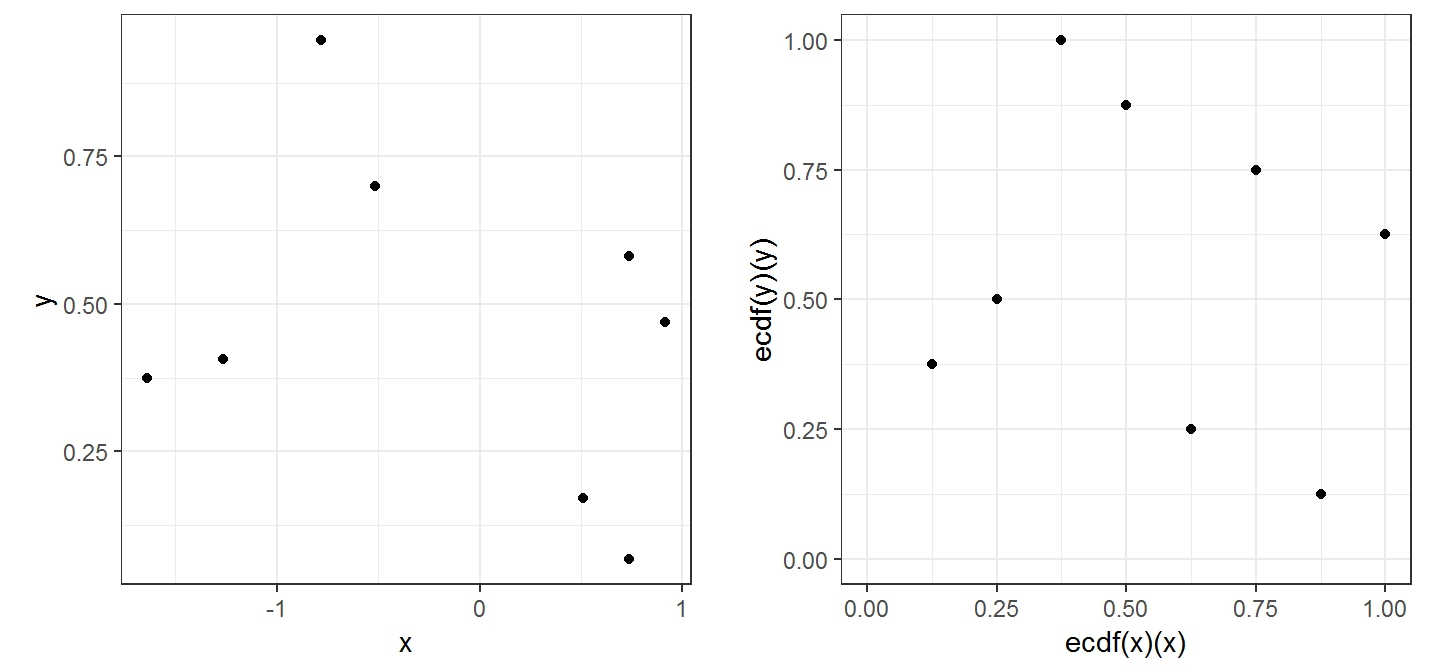similarly to the empirical distribution:

We start with a sample $$(x_1, y_1), \dots, (x_n, y_n)$$ and the pseudo - observations (normalized ranks).

## Empirical copula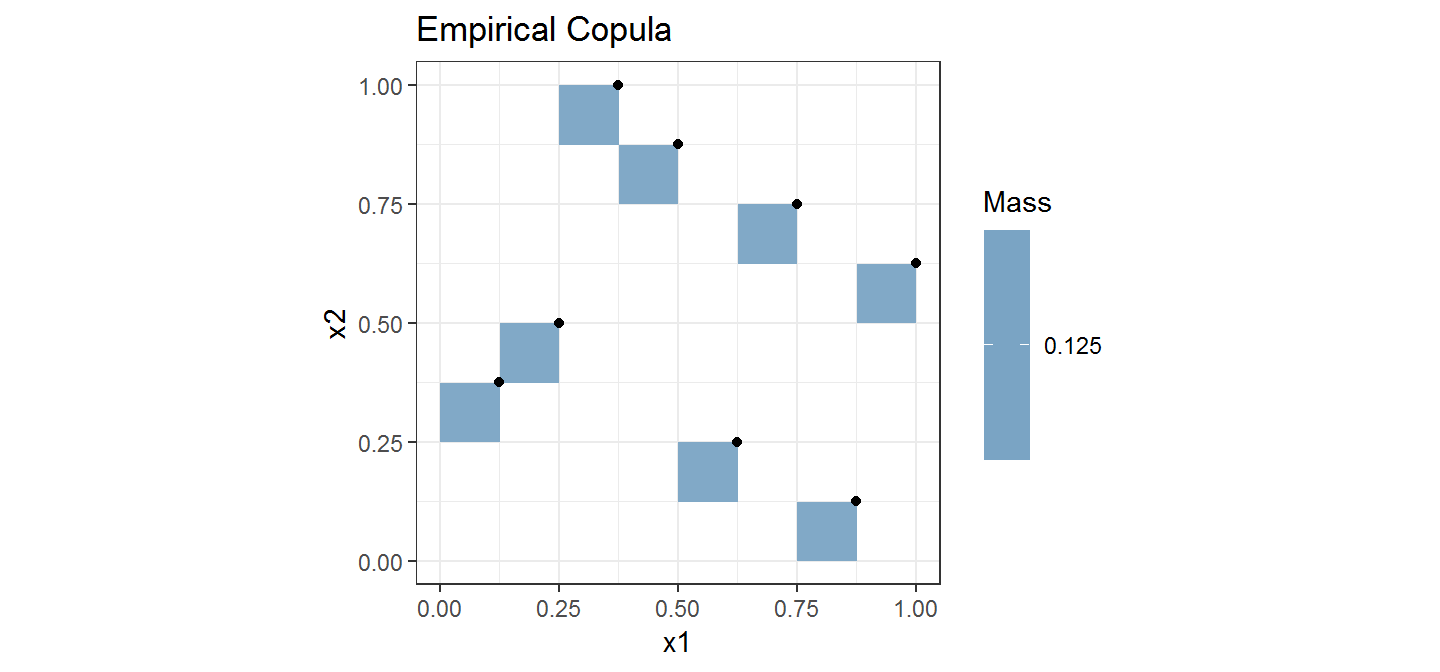And then proceed to construct an empirical copula as shown above.

## Empirical copula of our example

Let’s compute the empirical copula for our example: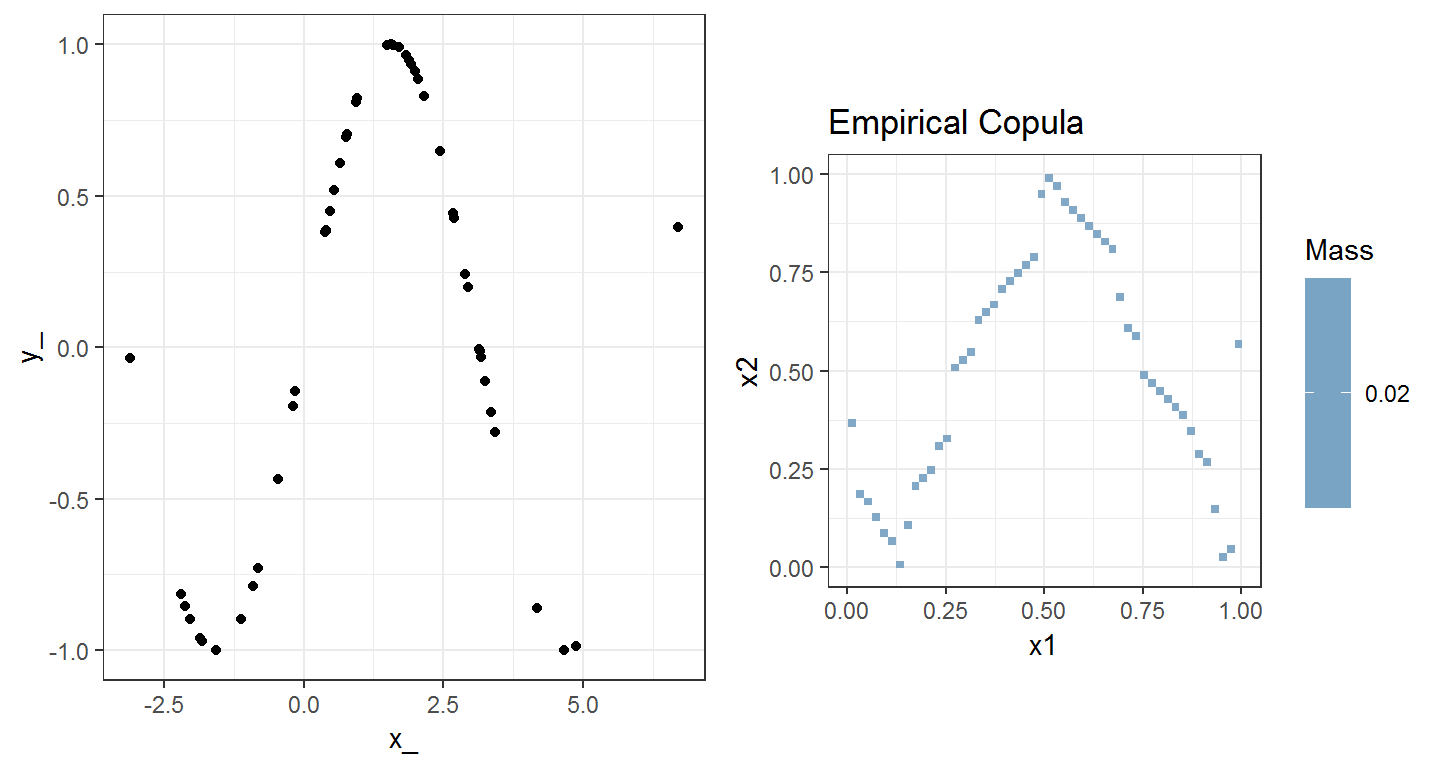## Limitations

We would wish that the empirical copula $$E_n$$ was a good estimate for the true underlying copula $$A$$.

Using the aforementioned metric $$D_1$$, this is not always the case.

We need to use a different estimator for $$A$$.

## Empirical checkerboard copula

We aggregate the empirical copula into what we call empirical checkerboard copula.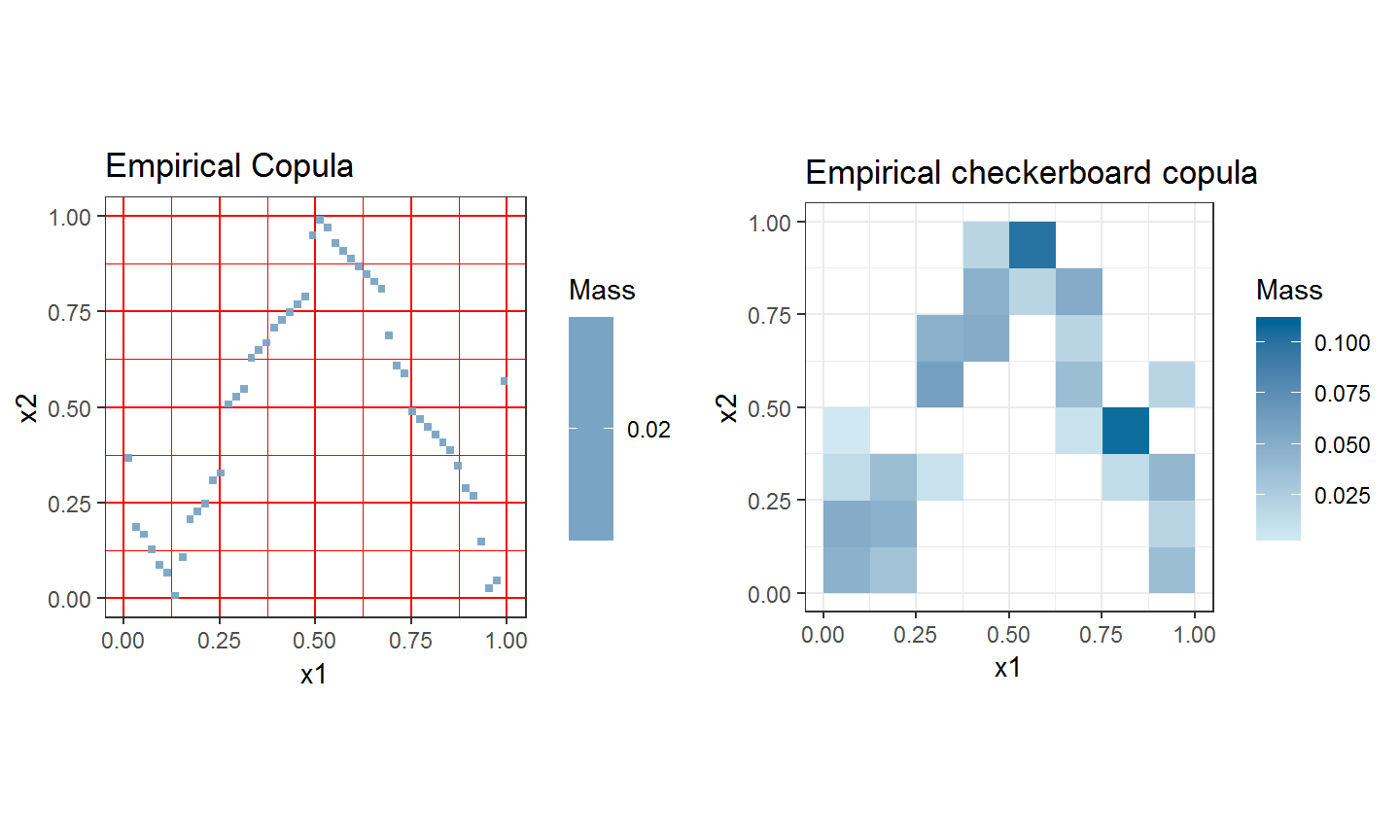Now, not only does the checkerboard copula $$\hat{C_n}$$ have nice analytical properties, it also is a good estimate for $$A$$ in our metric $$D_1$$, so $$D_1(\hat{C_n},A) \approx 0$$ for sufficiently large $$n$$.

## emp_c_copula()

The function emp_c_copula computes the mass-distribution of the empirical (checkerboard) copula, given a bivariate sample.

Arguments:

• X: a dataframe containing a bivariate sample

• smoothing: a logical indication whether the checkerboard copula is to be calculated

• resolution: an integer indicating the resolution of the checkerboard aggregation (the number of breaks in the grid)

## emp_c_copula()

The function emp_c_copula computes the mass-distribution of the empirical (checkerboard) copula, given a bivariate sample.

n = 50
x = rnorm(n,0,1)
y = runif(n,0,1)
df = data.frame(x,y)
emp_cop = emp_c_copula(df,smoothing = FALSE)
emp_check_cop = emp_c_copula(df,smoothing = TRUE,resolution = 20)

## plot_density()

The function plot_density plots the density/mass of the empirical (checkerboard) copula.

Arguments:

• mass_matrix: A squared matrix containing the mass distribution (output of emp_c_copula())

• density: A logical indication whether density or mass is plotted

## plot_density()

The function plot_density allows us to visualize copulae:

plot_density(emp_cop, density=FALSE)
plot_density(emp_check_cop, density=FALSE)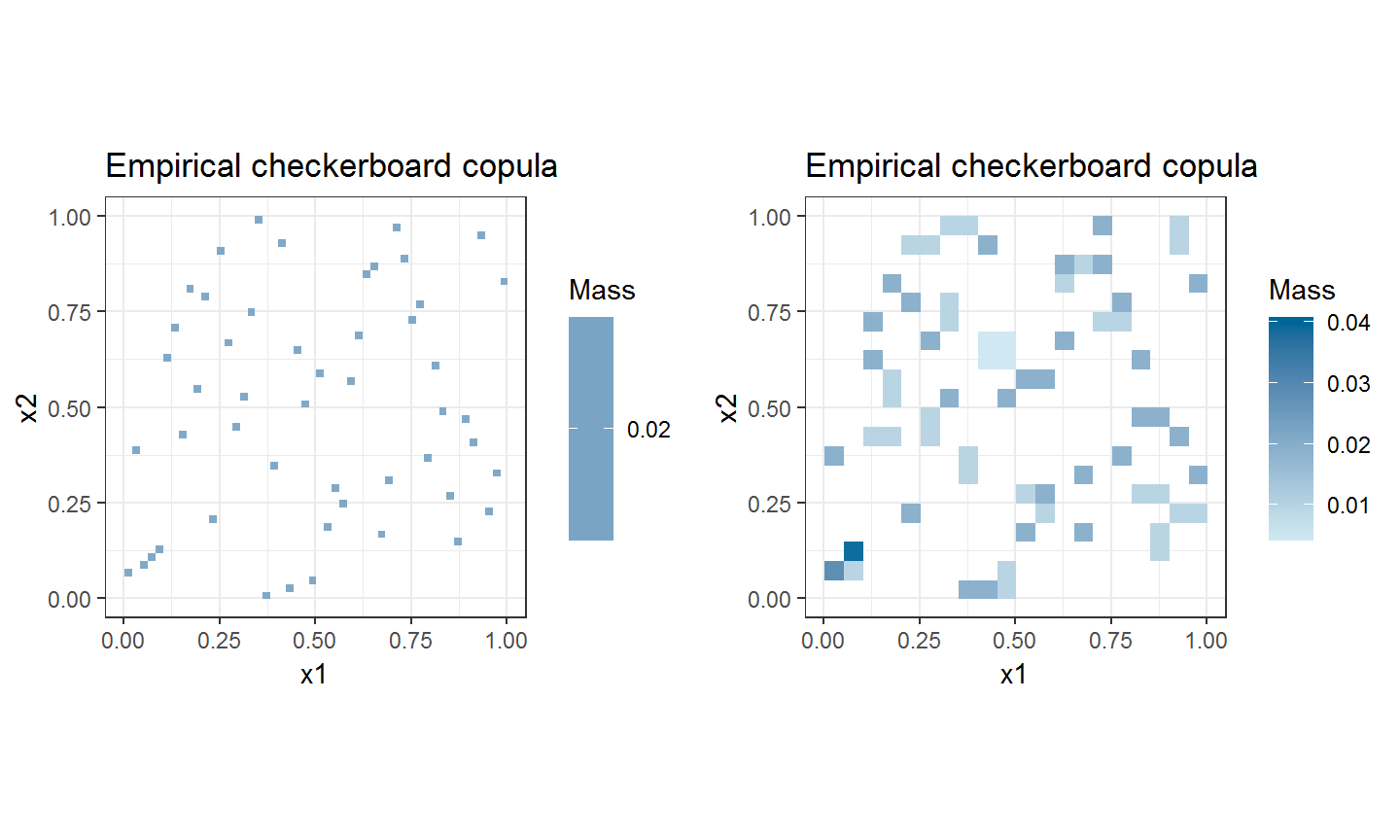## Exercise 1

Calculate the empirical copula – not the checkerboard copula – while having the argument smoothing=TRUE. (Hint: Resolution parameter)

Optional: Visualize this by plotting the pseudo observations of your generated sample into the mass-plot (Compare: slide 13,14).

Explain the differences in the following plots:

n=10
df = data.frame(x = rnorm(n), y = runif(n))
emp_cop = emp_c_copula(df, smoothing = FALSE)
emp_check_cop = emp_c_copula(df, smoothing = TRUE, resolution = 20)
plot_density(emp_cop, density = FALSE)
plot_density(emp_check_cop, density = FALSE)

## The dependency measure

Based on a metric $$D_1$$ for copulae, a dependency measure can be constructed in the following way: $q(A) := 3\cdot D_1(A,\Pi) \; \in [0,1]$ $$\Pi$$ denotes the product copula, which stems from two completely independet RVs.

In a way, we measure the “distance” to complete independece.

The function qad quantifies the asymmetric dependence of two RVs $$X$$ and $$Y$$. It achieves this by calculating the empirical checkerboard copula to estimate the dependency measure $$q$$.

Arguments:

• X: a dataframe containing a bivariate sample in two columns.

• resolution: resolution of the emp. copula

• premutation: a logical indicating, whether a permutated $$p$$-value is computed.

The function qad quantifies the asymmetric dependence of two RVs $$X$$ and $$Y$$. It achieves this by calculating the empirical checkerboard copula to estimate the dependency measure $$q$$.

There are multiple ways to see the results of the qad calculation:

• qad(X)$results ## qad Example 1 Back to our initial example: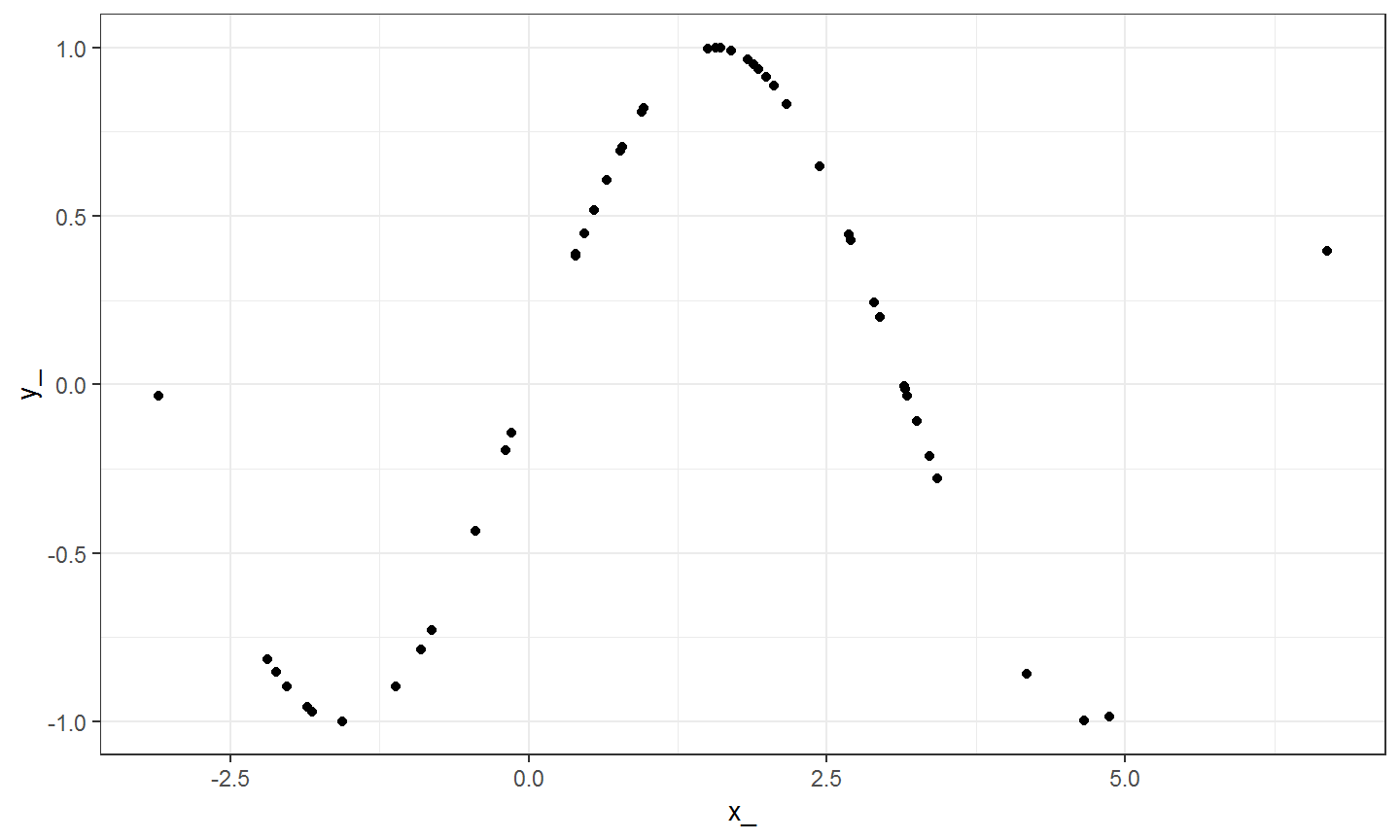## qad Example 1 We take a look at the mutual dependencies. mod = qad(X, print = FALSE) coef(mod, select = c("q(x1,x2)","q(x2,x1)","mean.dependence", "asymmetry")) ## q(x1,x2) q(x2,x1) mean.dependence asymmetry ## 0.7885979 0.4193818 0.6039898 0.3692161 We see that $$X$$ predicts $$Y$$ better than $$Y$$ predicts $$X$$. ## qad Example 2 n = 200 x2 = runif(n,-2,4) y2 = exp(x2) df = data.frame(x2,y2) model = qad(df, print = FALSE, permutation = TRUE) model$results
##                        coef p.values
## 1        q(x1,x2) 0.9608946        0
## 2        q(x2,x1) 0.9608946        0
## 3 mean.dependence 0.9608946        0
## 4 asymmetry       0.0000000        1

With the function pairwise.qad, we can apply the qad function on each pair of columns of the given dataframe. Other arguments are the same as for qad.

n = 1000
x = runif(n,0,1)
y = x^2+rnorm(n,0,1)
z = runif(n,0,1)
df = data.frame(x,y,z)

model = pairwise.qad(df, permutation = TRUE)
##  "Process..."
##  "Process: 1 / 3"
##  "Process: 2 / 3"

We can plot the results as a heatmap:

heatmap.qad(model, select = "dependence", fontsize = 8,
significance = TRUE)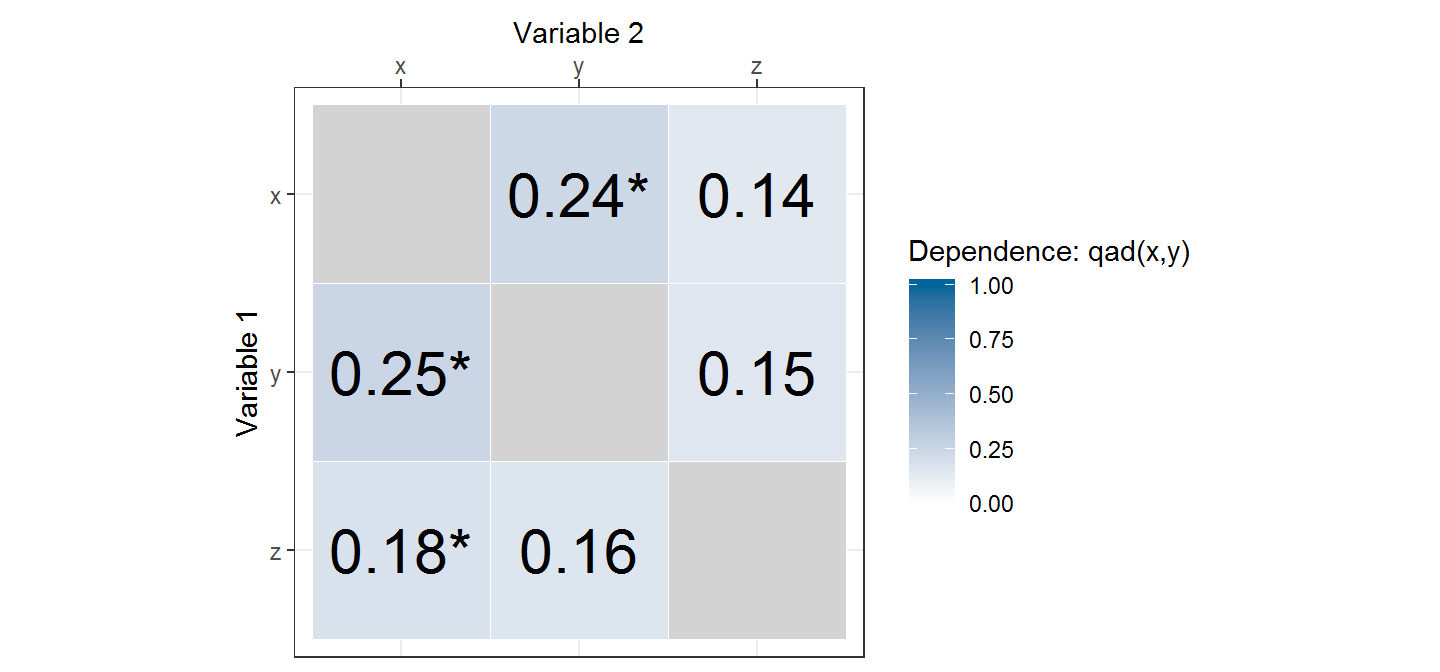Arguments:

• select: dependence value to be plotted: “dependence”, “mean.dependence” or “asymmetry”

• significance: logical; if p-values were calculated, marks the significant dependency values with a star

• sign.level: manually set significance level (default: 0.05)

## Exercise 2

and sample 1000 observations.

• Examine which columns could have interesting dependencies.

• Why does this not make sense for all columns?

• Visualize the dependencies for the relevant columns. Justify why you chose them.

plot(mod, addSample = TRUE, copula = TRUE)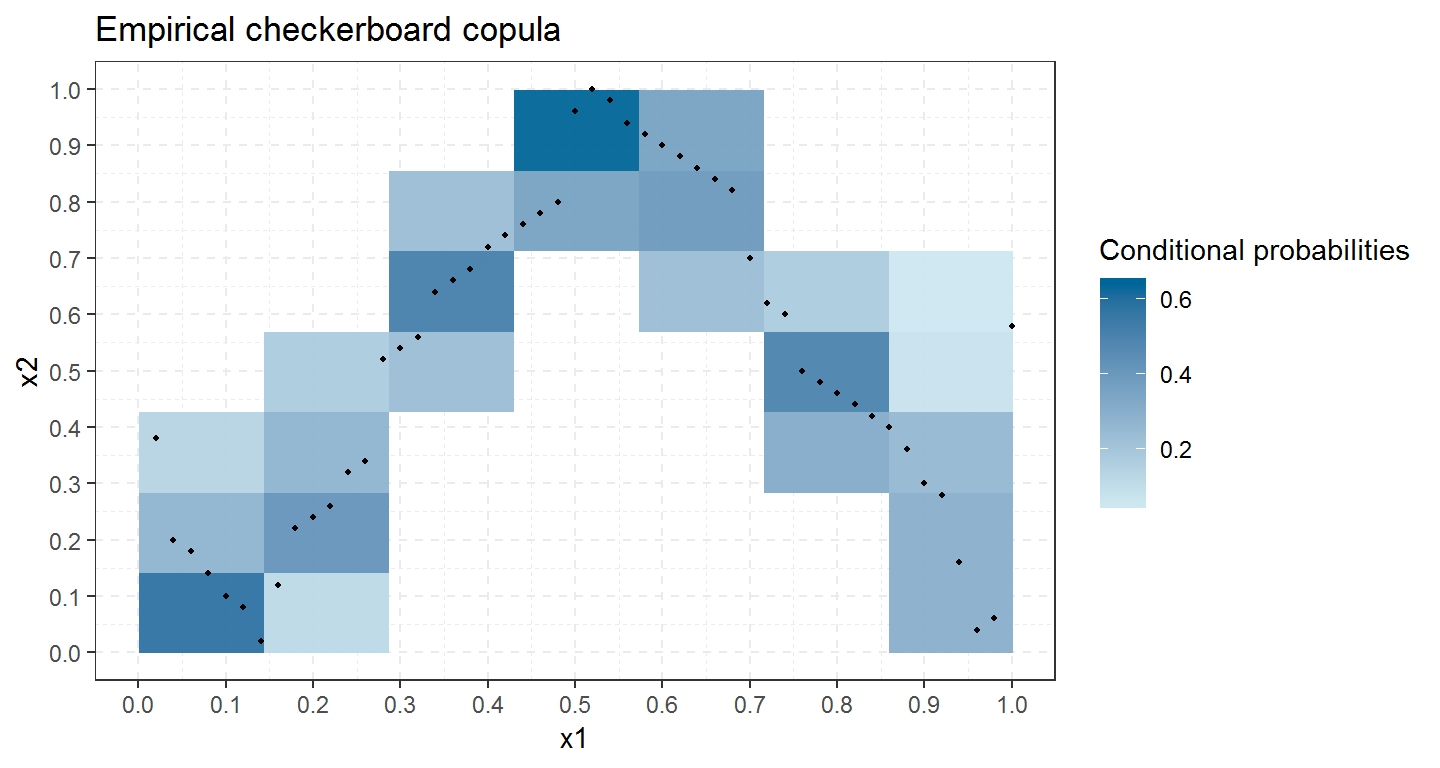plot(mod, addSample = TRUE)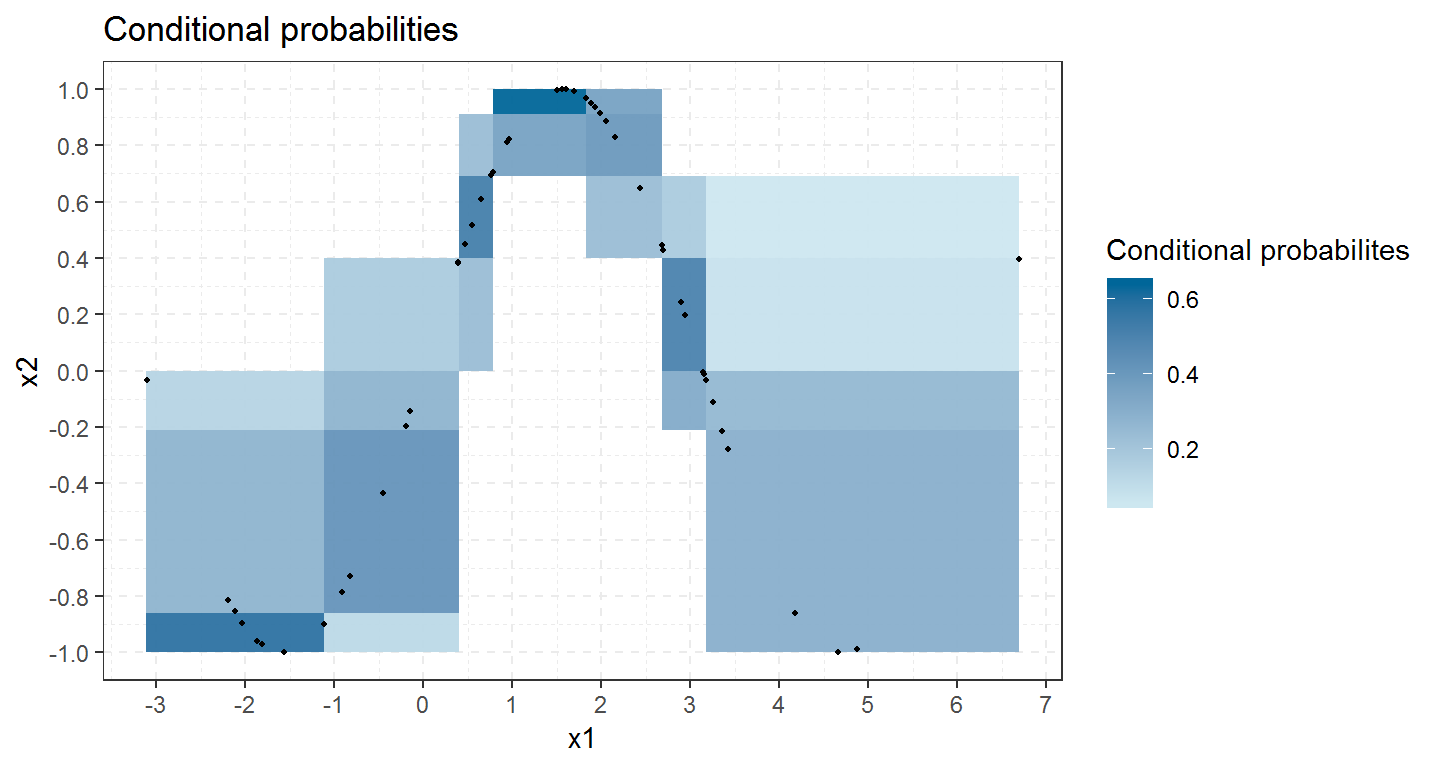The function predict.qad() predicts the probabilities to end up in specific intervals given $$x$$ or $$y$$ values.

The prediciton can be computed in the copula or the retransformed data setting.

predict(mod, values = 1.42, conditioned = "x1", nr_intervals = 5)
##      (-1,-0.79] (-0.79,-0.02] (-0.02,0.43] (0.43,0.82] (0.82,1]
## 1.42          0             0            0       0.216    0.784

## Exercise 3

• Create a dummy dataset: n = 100 observations of a uniformly distributed random variable and the corresponding square values.

• Create a new qad object and use the plot function to visualize the copula.

• Use the predict.qad method and sum up the probabilities for one strip (horizontal or vertical) for one value.

## cci - Conditional Confidence Interval

We can also use the qad metric to test hypothesis:

cci provides a confidence interval for independence (for the qad measure).

We can compare the qad-value to the interval boundaries and check if the null hypothesis of independence holds or has to be rejected.

## cci

We calculate our boundaries

c = cci(n, alternative = "one.sided")

and check, whether the calculated dependence for our example lies between them.

if(coef(mod, select = 'q(x1,x2)') %in% c){
print('Accept H0')
}else{
print('Reject H0')
}

## cci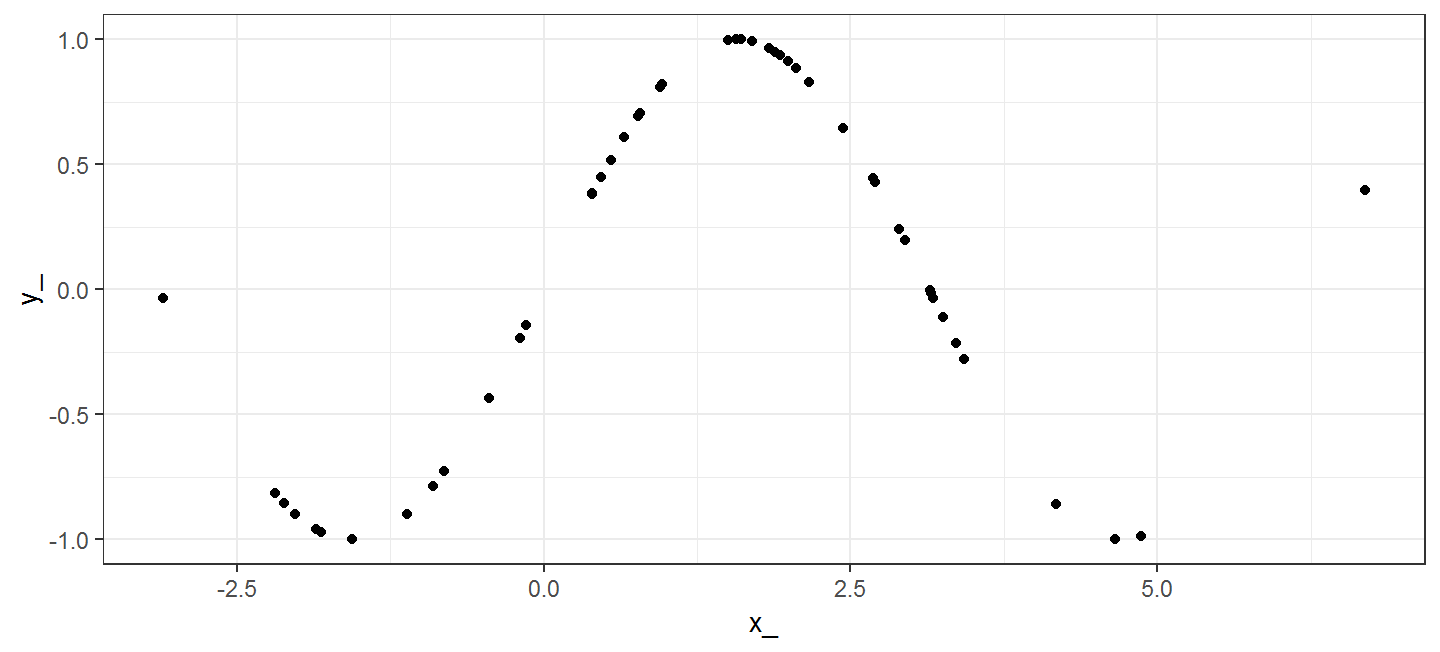And indeed, for our example, we reject the hypothesis of independence:

##  "q(x1,x2) not in [ 0 , 0.1813481327652 ]"
##  "Reject H0"

## cciSimilarly for the other direction:

##  "q(x2,x1) not in [ 0 , 0.1813481327652 ]"
##  "Reject H0"

## Final Exercise 4:

Take a look at the attitude dataset:

Find out the two attributes with the highest (one sided) dependency. Provide respective plots.

Are those dependencies symmetric? Argue by providing p-values for asymmetry (hint: do not to calculate the p-values for all pairs, only the chosen two)

Reject or Accept the hypothesis of independence for your chosen pairs.